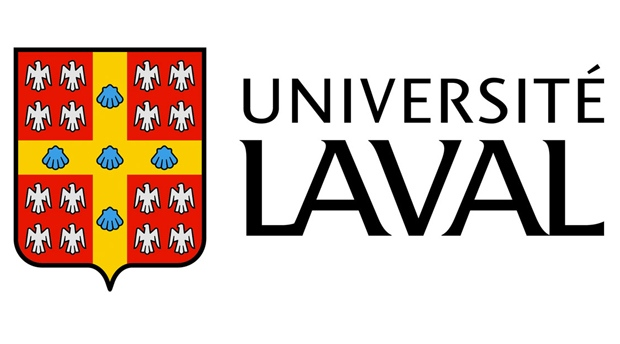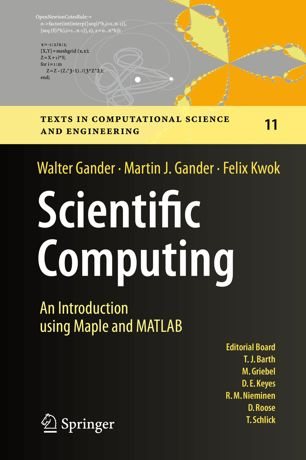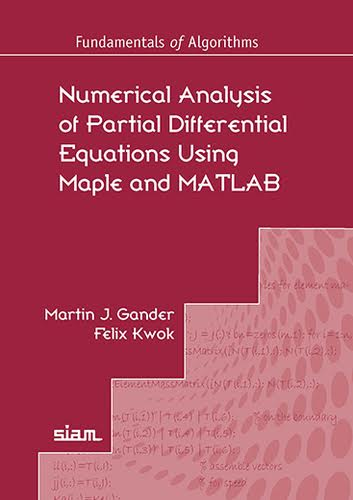Felix Kwok Département de mathématiques et de statistique Université Laval## Books

### Scientific Computing: An Introduction Using Maple and MATLABby Walter Gander, Martin J. Gander and Felix Kwok Published by Springer Series: Texts in Computational Science and Engineering 11 ISBN: 978-3-319-04324-1 Year: 2014 DOI: 10.1007/978-3-319-04325-8 Equal emphasis on theory, symbolic computation and numerical algorithms Scientific Computing is the study of how to use computers effectively to solve problems that arise from the mathematical modeling of phenomena in science and engineering. It is based on mathematics, numerical and symbolic/algebraic computations and visualization. This book serves as an introduction to both the theory and practice of scientific computing, with each chapter presenting the basic algorithms that serve as the workhorses of many scientific codes: we explain both the theory behind these algorithms and how they must be implemented in order to work reliably in finite-precision arithmetic. The book includes many programs written in Matlab and Maple - Maple is often used to derive numerical algorithms, wheras Matlab is used to implement them. The theory is developed in such a way that students can learn by themselves as they work through the text. Each chapter contains numerous examples and problems to help readers understand the material 'hands-on'. Download runnable codes and errata here.

### Numerical Analysis of Partial Differential Equations Using Maple and MATLABby Martin J. Gander and Felix Kwok Published by SIAM Series: Fundamentals of Algorithms 12 ISBN: 978-1-611-97530-7 Year: 2018 DOI: 10.1137/1.9781611975314 This book provides an elementary yet comprehensive introduction to the numerical solution of partial differential equations (PDEs). Used to model important phenomena, such as the heating of apartments and the behavior of electromagnetic waves, these equations have applications in engineering and the life sciences, and most can only be solved approximately using computers. Numerical Analysis of Partial Differential Equations Using Maple and MATLAB provides detailed descriptions of the four major classes of discretization methods for PDEs (finite difference method, finite volume method, spectral method, and finite element method); self-contained convergence proofs for each method, using the tools and techniques required for the general convergence analysis but adapted to the simplest setting to keep the presentation clear and complete; and runnable MATLAB© code for each of the discretization nmethods and exercises. This book is intended for advanced undergraduate and early graduate students in numerical analysis and scientific computing and researchers in related fields. It is appropriate for a course on numerical methods for partial differential equations. Download runnable codes and errata here.